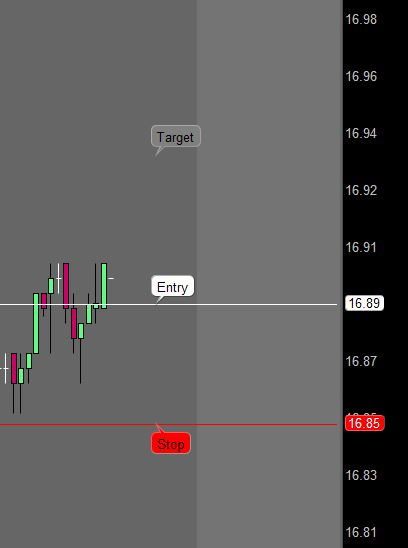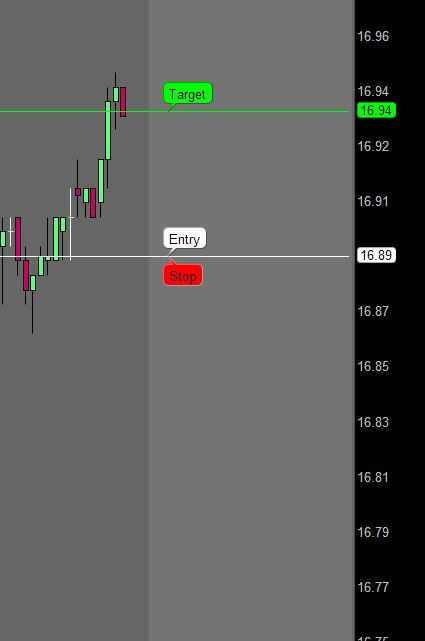# Adjust Stop Loss if Target Threshold is Reached

#### hammerschloggen

##### New member
I am working on a strategy that is functioning properly up to a certain point, but I want to be able to adjust my Stop Loss if the High ever breaks a Target Threshold.
The primary avenue I have explored is to use the GetValue() and GetMaxValueOffset() to check if the High has ever been greater than the Target since the trade was made. Unfortunately, I have to use a counter to do so and since the count number is dynamic it does not work with the GetMaxValueOffset() function.
Any suggestions would be great. Assume ExitCondition has some pre-defined criteria.

Code:
``````def Target = EntryPrice() + EntryPrice() * 0.05;
def StopLoss = EntryPrice() - EntryPrice() * 0.05;

def Count = if !isNaN(EntryPrice()) then Count + 1 else 0;
def OverTarget = GetValue(high, GetMaxValueOffset(high, Count), Count) >= Target;

def ExitPrice = if OverTarget then EntryPrice() else StopLoss;
AddOrder(OrderType.SELL_TO_CLOSE, ExitCondition, ExitPrice, tickcolor = Color.RED, arrowcolor = Color.RED, name = "Exit" + BarNumber());``````

#### SleepyZ

##### Well-known member
I am working on a strategy that is functioning properly up to a certain point, but I want to be able to adjust my Stop Loss if the High ever breaks a Target Threshold.
The primary avenue I have explored is to use the GetValue() and GetMaxValueOffset() to check if the High has ever been greater than the Target since the trade was made. Unfortunately, I have to use a counter to do so and since the count number is dynamic it does not work with the GetMaxValueOffset() function.
Any suggestions would be great. Assume ExitCondition has some pre-defined criteria.

Code:
``````def Target = EntryPrice() + EntryPrice() * 0.05;
def StopLoss = EntryPrice() - EntryPrice() * 0.05;

def Count = if !isNaN(EntryPrice()) then Count + 1 else 0;
def OverTarget = GetValue(high, GetMaxValueOffset(high, Count), Count) >= Target;

def ExitPrice = if OverTarget then EntryPrice() else StopLoss;
AddOrder(OrderType.SELL_TO_CLOSE, ExitCondition, ExitPrice, tickcolor = Color.RED, arrowcolor = Color.RED, name = "Exit" + BarNumber());``````
See if this helps: First image is before Target is met. Second image is moving stop to entry when Target is met.Code:
``````#Example_AutoAdjust_StopLoss
#Sleepz
def cond        = ExpAverage(close, 3) crosses above ExpAverage(close, 8);
def entryprice  = if cond then close else entryprice;
def entrybar    = if cond then BarNumber() else Double.NaN;
def targetprice = entryprice + .05;

plot targetmet   = HighestAll(if BarNumber() > HighestAll(entrybar) and high crosses above targetprice then targetprice else Double.NaN);
targetmet.SetDefaultColor(Color.GREEN);

plot ep = HighestAll(if BarNumber() == HighestAll(entrybar) then entryprice else Double.NaN);
ep.SetDefaultColor(Color.WHITE);

plot stoploss = HighestAll(if BarNumber() < HighestAll(entrybar) then Double.NaN else if !IsNaN(targetmet) then entryprice else entryprice - .04);
stoploss.SetDefaultColor(Color.RED);

input bubblemover = 5;
def b  = bubblemover;
def b1 = b + 1;
AddChartBubble(IsNaN(close[b]) and !IsNaN(close[b1]), ep[b1], "Entry", Color.WHITE, yes);
AddChartBubble(IsNaN(close[b]) and !IsNaN(close[b1]), stoploss[b1], "Stop", Color.RED, no);
AddChartBubble(IsNaN(close[b]) and !IsNaN(close[b1]), targetprice[b1], "Target", if !IsNaN(targetmet[b1]) then Color.GREEN else Color.GRAY, yes);``````

•scott69 and RickAns

#### hammerschloggen

##### New member
Thanks @SleepyZ
I ran this with a section of my script and it seems to work.

•SleepyZ